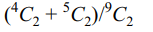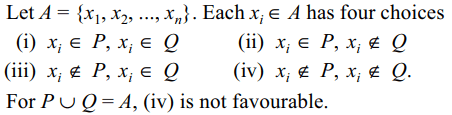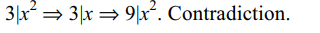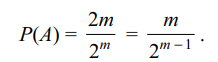## Probability Questions and Answers Part-8

1. Suppose X ~ B(n, p) and P(X = 3) = P(X = 5). If p > 1/2, then
a) $n\leq 7$
b) n > 8
c) $n\geq 9$
d) $n\geq 10$

Explanation:2. In three throw of a pair dice, the probability throwing doublets not more than twice is
a) 1/6
b) 5/72
c) 215/216
d) 7/128

Explanation:3. A bag contains 4 brown and 5 white socks. A man pulls two socks at random without replacement. The probability that the man gets both the socks of the same colour is
a) 5/108
b) 1/6
c) 5/18
d) 4/9

Explanation:4. A is a set containing n elements. Two subsets P and Q of A are chosen at random. (P and Q may have elements in common). The probability that $P\cup Q\neq A$   is
a) $\left(3/4\right)^{n}$
b) 1/4n
c) $^{n}C_{2}/2^{n}$
d) $1-\left(3/4\right)^{n}$

Explanation:5. Three of the six vertices of a regular hexagon are chosen at random. The probability that the triangle with these vertices is not equilateral is
a) 1/2
b) 1/5
c) 9/10
d) 1/20

Explanation: Find probability of the complement event.
9/10

6. A positive integer is chosen at random. The probability that the sum of the digits of its square is 39 is
a) 1/39
b) 2/39
c) 1/11
d) 0

Explanation:7. A lottery sells $n^{2}$ tickets and declares n prizes. If a man purchases n tickets, the probability of his winning at least one prize is
a) $\left(n^{2}-n\right)!/\left(n^{2}\right)!$
b) 1/2n
c) $\left(n-1\right)!^{2}/\left(n^{2}\right)!$
d) none of these

Explanation:8. Let x be a non-zero real number. A determinant is chosen from the set of all determinants of order 2 with entries x or – x only. The probability that the value of the determinant is non-zero is
a) 3/16
b) 1/4
c) 1/2
d) 1/8

Explanation:9. A die is rolled three times. The probability of getting a number larger than the previous number each time is
a) 5/72
b) 5/54
c) 13/216
d) 1/18b) $m/2^{m-1}$
d) $1/2^{m-1}$